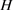﻿ 具有自中心化正规子群的有限群的Coleman自同构

# 具有自中心化正规子群的有限群的Coleman自同构Coleman Automorphisms of Finite Groups with a Self-Centralizing Normal Subgroup

Abstract: Let G be a finite group with a self-centered normal subgroup N. In this note, we studied the effects of the properties of the self-centered normal subgroup N on the structure of the Coleman outer automorphism group of G and proved that all Coleman automorphisms of G are inner when N is under some conditions. Such Coleman automorphisms play an important role in the research of normalizer problem for integral group rings.

1. 引言

$G$ 为有限群， $\sigma$$G$ 的一个自同构，如果 $\sigma$$G$ 的任意一个 $Sylow$ 子群上的限制等于 $G$ 的某个内自同构在其上的限制，则称 $\sigma$$G$ 的一个Coleman自同构。 $G$ 的所有的Coleman自同构组成了 $Aut\left(G\right)$ 的一个子群，记为 $Au{t}_{Col}\left(G\right)$ 。显然 $Inn\left(G\right)⊴Au{t}_{Col}\left(G\right)$ ，称商群 $Au{t}_{Col}\left(G\right)/Inn\left(G\right)$$G$ 的Coleman外自同构群，记为 $Ou{t}_{Col}\left(G\right)$ 。在文献  中，Hertweck和Kimmerle证明了 $Ou{t}_{Col}\left(G\right)$ 是交换群，给出了 $Ou{t}_{Col}\left(G\right)$${p}^{\prime }$ -群的一些充分条件。在文献  中，海进科等研究了因子群的性质对有限群 $G$ 的Coleman外自同构群的影响，也给出了 $Ou{t}_{Col}\left(G\right)$${p}^{\prime }$ -群的一些充分条件。在文献  中，李正兴等证明了具有唯一非平凡正规子群的有限群 $G$ 的Coleman自同构为内自同构。Antwerpen在文献  中研究了具有自中心化特征单的正规子群的有限群的Coleman自同构，证明了这样的群的Coleman自同构也为内自同构。在文献  中，赵文英等人也研究了具有自中心化正规子群的有限群的Coleman自同构，并给出了 $Ou{t}_{Col}\left(G\right)=1$ 的一些充分条件。其它有关Coleman自同构方面的结果可参见文献   。

$G$ 是一个有限群，我们用 $ZG$ 表示 $G$ 在整群环 $Z$ 上的整群环，用 $U\left(\text{Z}G\right)$ 表示 $ZG$ 的单位群，用 ${N}_{U\left(\text{Z}G\right)}\left(G\right)$ 表示 $G$ 在单位群 $U\left(\text{Z}G\right)$ 中的正规化子。对任意 $u\in {N}_{U\left(\text{Z}G\right)}\left(G\right)$ ，用 ${\phi }_{u}$ 表示由 $u$ 通过共轭诱导的 $G$ 的自同构，记 $Au{t}_{Z}\left(G\right)\text{=}\left\{{\phi }_{u}|u\in {N}_{U\left(ZG\right)}\left(G\right)\right\}$ ，令 $Ou{t}_{Z}\left(G\right)=Au{t}_{Z}\left(G\right)/Inn\left(G\right)$ 。由文献  中的问题43知， $G$ 具有正规化子性质当且仅当 $Ou{t}_{Z}\left(G\right)=1$ 。由著名的Coleman引理知 $Ou{t}_{Z}\left(G\right)\le Ou{t}_{Col}\left(G\right)$ ，所以只要能够证明 $Ou{t}_{Col}\left(G\right)=1$ ，那么 $G$ 具有正规化子性质，这促使人们去研究什么样的有限群 $G$ 满足 $Ou{t}_{Col}\left(G\right)=1$

$G$ 为完全群，如果 $\mathcal{Z}\left(G\right)=1$$Aut\left(G\right)=Inn\left(G\right)$ 。称 $H$ 为几乎单群，如果存在非交换单群 $G$ ，使得 $G\le H\le Aut\left(G\right)$ 。称 $G$ 为有限群 $S$ 和有限群 $H$ 的圈积，如果 $G$${S}^{|H|}$$H$ 的半直积，其中 ${S}^{|H|}=S×\cdots ×S$$|H|$$S$ 的直积，记为 $G=SwrH$ 。本文我们继续研究自中心化正规子群 $N$ 的性质对 $G$ 的Coleman外自同构群结构的影响，证明了下面主要结果：

2. 定理的证明

1) $G$ 中包含的唯一最小正规子群；

2) ${H}^{G}=〈{h}^{g}|h\in H,g\in G〉$

${\sigma }_{1}{}^{tm}={\left(\sigma conj\left({h}^{-1}\right)\right)}^{tm}\in {\left(\sigma Inn\left(G\right)\right)}^{tm}={\sigma }^{tm}Inn\left(G\right)$

${x}^{-1}nx={\left({x}^{-1}nx\right)}^{\beta }={\left({x}^{-1}\right)}^{\beta }{n}^{\beta }{x}^{\beta }={\left({x}^{-1}\right)}^{\beta }n{x}^{\beta }$

${x}^{-1}nx={\left({x}^{-1}nx\right)}^{{\rho }_{2}}={\left({x}^{-1}\right)}^{{\rho }_{2}}{n}^{{\rho }_{2}}{x}^{{\rho }_{2}}={\left({x}^{-1}\right)}^{{\rho }_{2}}n{x}^{{\rho }_{2}}$

$Q$$S$$Sylow$ $q$ -子群，则 ${Q}^{n}$${S}^{n}$$Sylow$ $q$ -子群。由 $Sylow$ 定理知，存在 $G$$Sylow$ $q$ -子群 $P$ ，使得 ${Q}^{n}=Q×\cdots ×Q\le P$

${z}^{{\rho }_{2}}={\left({h}^{-1}gh\right)}^{{\rho }_{2}}={\left({h}^{-1}\right)}^{{\rho }_{2}}{g}^{{\rho }_{2}}{h}^{{\rho }_{2}}={\left({h}^{-1}\right)}^{{\rho }_{2}}g{h}^{{\rho }_{2}}$

${z}^{{\rho }_{2}}={\left({h}^{-1}\right)}^{{\rho }_{2}}g{h}^{{\rho }_{2}}={x}_{n}{}^{-1}···{x}_{2}{}^{-1}{x}_{1}{}^{-1}g{x}_{1}{x}_{2}\cdots {x}_{n}\in {S}_{j}$

${\rho }_{2}|{}_{{S}_{j}}\in Aut\left({S}_{j}\right)$ 。这就证明了 ${\rho }_{2}|{}_{S}\in Aut\left(S\right)$ ，因此 ${\rho }_{2}$$S$$q$ -中心自同构。

${x}^{-1}yx={\left({x}^{-1}yx\right)}^{{\rho }_{2}}={\left({x}^{-1}\right)}^{{\rho }_{2}}{y}^{{\rho }_{2}}{x}^{{\rho }_{2}}={\left({x}^{-1}\right)}^{{\rho }_{2}}y{x}^{{\rho }_{2}}$

① 如果 $q=p$ ，则 ${\rho }_{2}{|{}_{P}=id|}_{P}$ 。又 ${\rho }_{2}{|{}_{{S}^{n}}=id|}_{{S}^{n}}$${\rho }_{2}{|{}_{G/{S}^{n}}=id|}_{G/{S}^{n}}$${\rho }_{2}$ ${\rho }_{2}={\rho }_{1}{}^{k}={\left(\sigma conj\left({h}^{-1}\right)\right)}^{k}$$p$ -方幂阶的Coleman自同构，由引理3知， ${\rho }_{2}\in Inn\left(G\right)$ 。因为，由引理7可知， $\sigma conj\left({h}^{-1}\right)\in Inn\left(G\right)$ ，故 $\sigma \in Inn\left(G\right)$

② 如果 $q\ne p$ ，则对任意 $u\in G$ ，存在 $v\in {S}^{n}$ ，使得 ${u}^{{\rho }_{2}}=uv$ 。因为 ${\rho }_{2}$$p$ -方幂阶的，所以可设 $o\left({\rho }_{2}\right)={p}^{r}$ ，其中 $r$ 为非负整数，那么 $u={u}^{{\rho }_{2}{}^{{p}^{r}}}=u{v}^{{p}^{r}}$ ，因此 ${v}^{{p}^{r}}=1$ ，而 ${S}^{n}$$q$ -群，所以 $v=1$ 。由 $u$ 的任意性可知 ${\rho }_{2}=id$ 。又因为 ${\rho }_{2}={\rho }_{1}{}^{k}={\left(\sigma conj\left({h}^{-1}\right)\right)}^{k}$ ，由引理7可知， $\sigma conj\left({h}^{-1}\right)\in Inn\left(G\right)$ ，故 $\sigma \in Inn\left(G\right)$

NOTES

*通讯作者。

 Hertweck, M. and Kimmerle, W. (2002) Coleman Automorphisms of Finite Groups. Mathematische Zeitschrift, 242, 203-215.
https://doi.org/10.1007/s002090100318

 Hai, J.K. and Li, Z.X. (2014) On Coleman Outer Automorphism Groups of Finite Groups. Acta Mathematica Scientia, 34, 790-796.
https://doi.org/10.1016/S0252-9602(14)60049-7

 Li, Z.X., Hai, J.K. and Yang, S.X. (2014) Coleman Automorphisms of Finite Groups with a Unique Nontrivial Normal Subgroup. Journal of Mathematical Research with Applications, 34, 301-306.

 Antwerpen, A.V. (2018) Coleman Automorphisms of Finite Groups and Their Minimal Normal Subgroups. Journal of Pure and Applied Algebra, 222, 3379-3394.
https://doi.org/10.1016/j.jpaa.2017.12.013

 赵文英, 海进科. 关于有限内幂零群和Frobenius群的Coleman自同构[J]. 山东大学学报(理学版), 2017, 52(10): 4-6.

 Hai, J.K. and Zhu, Y.X. (2018)On Coleman Automorphisms of Extensions of Finite Quasinilpotent Groups by Some Groups. Algebra Colloquium, 25, 181-188.
https://doi.org/10.1142/S1005386718000123

 Hai, J.K., Ge S.B. and He, W.P. (2017) The Nor-malizer Property for Integral Group Rings of Holomorphs of Finite Nilpotent Groups and the Symmetric Groups. Journal of Algebra and Its Applications, 16, 39-44.
https://doi.org/10.1142/S0219498817500256

 Sehgal, S.K. (1993)Units in Integral Group Rings. Longman Scientific and Technical Press, Harlow.

 Rose, J.S. (1978) A Course on Group Theory. Cambridge University Press, Cambridge.

Top